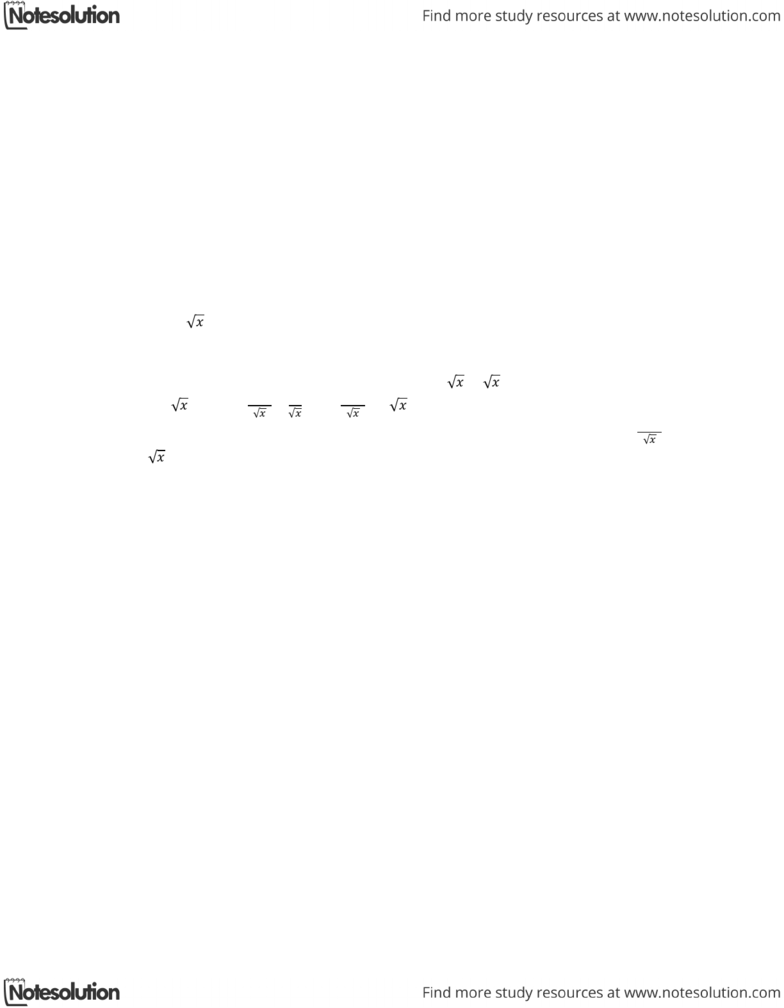Class Notes (1,100,000)
CA (630,000)
UTSG (50,000)
MAT (4,000)
MAT136H1 (900)
all (200)
Lecture

# 9.5 Linear Equations Question #1 (Easy)

Department
Mathematics
Course Code
MAT136H1
Professor
all

Page:
of 19.5 Differential Equations
Linear Equations
Question #1 (Easy): Determining if the Differential Equation is Linear
Strategy
Standard linear form is    
Any differential equation when rearranged is put into this form is linear.
Sample Question
Determine if the differential equation is linear.
    
Solution
When the differential equation is rearranged:     ;    ; dividing
both sides by :   

  
 .
Therefore this is in the form of    , where can be identified as  
and
 .